Triangles

Triangles are a three-sided polygon that consists of three edges and three vertices. The most important property of a triangle is that the sum of the internal angles of a triangle is equal to 180o. There are basically 6 different types of triangles, which we are going to discuss in the latter part. There are various formulas such as perimeter and area defined for the triangle. Also, its properties are based on its sides and angles. Here, in this article, we will learn the definition, types, properties, Heron’s Formula to find the area along with examples.

Definition of Triangle

As we discussed in the introduction, a triangle is a type of polygon, which has three sides joint end to end and three angles, formed between two sides. This is one of the important parts of geometry. Some of the major concepts such as Pythagoras theorem and trigonometry are dependent on triangle properties. Let us learn now its types.

On the basis of length of the sides. triangles are classified into three categories:

1. Scalene Triangle
2. Isosceles Triangle
3. Equilateral Triangle

On the basis of measurement of the angles, triangles are classified into three categories:

1. Acute Angle Triangle
2. Right Angle Triangle
3. Obtuse Angle Triangle

Below given is a triangle having 3 sides and three edges numbered as 0,1,2.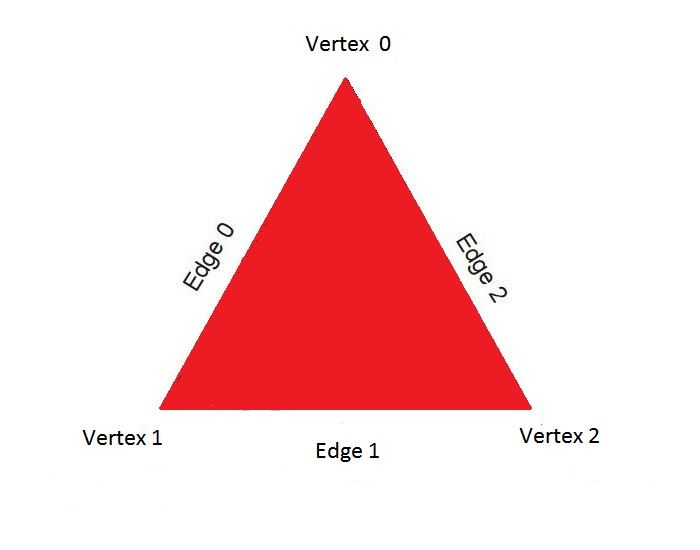Properties of Triangle

Each and every shape and figure in Maths have some properties which distinguish them from each other. Let us discuss here some of the properties of triangles.

1. Sum of Angles of a Triangle is always 180 degrees.
2. The exterior angles of a triangle always add up to 360 degrees.
3. The sum of the lengths of any two sides of a triangle is greater than the length of the third side. Similarly, the difference between the lengths of any two sides of a triangle is less than the length of the third side.
4. The shortest side is always opposite to the smallest interior angle. Similarly, the longest side is always opposite to the largest interior angle.

Types of Triangles

Scalene Triangle

This type of triangle has all its three edges of different length. Due to this, the three angles are also different from each other.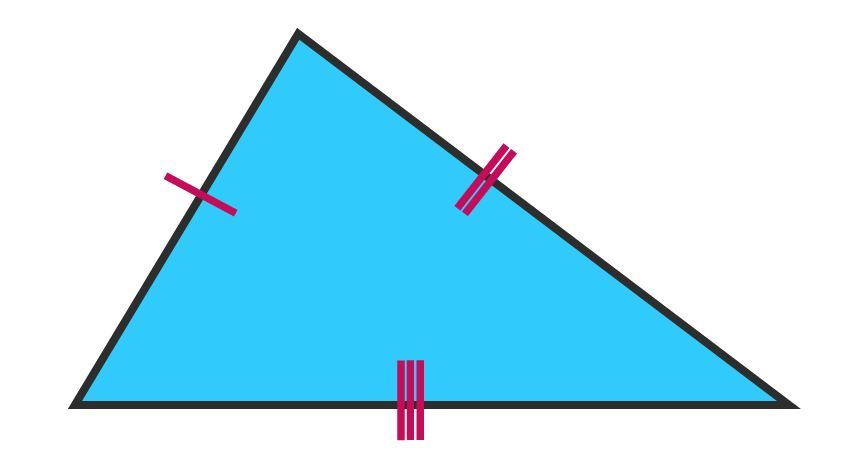Isosceles Triangle

This type of triangle has two equal sides. Also, the two angles, opposite to the two equal sides, are also equal to each other. This fact also supports the famous isosceles triangle theorem.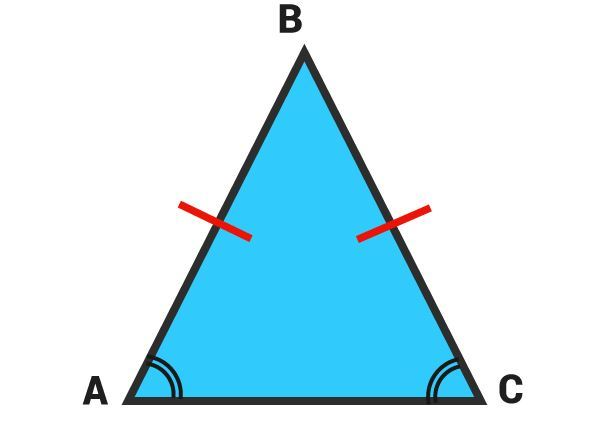Equilateral Triangle

This type of triangle has all three sides equal to each other. Due to this all the internal angles are of equal degrees ie. each of the angles is 60°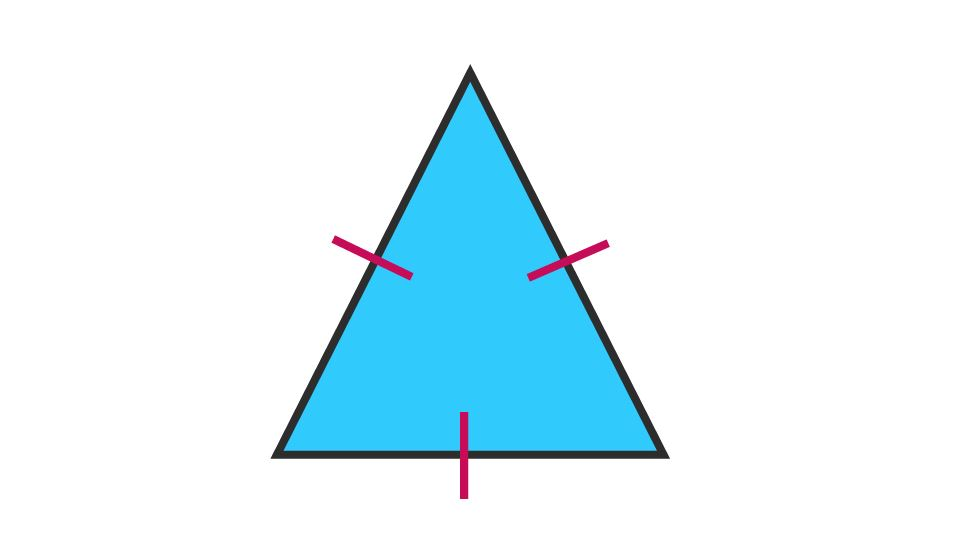Acute Angle Triangle

This triangle has all of its angles less than 90°.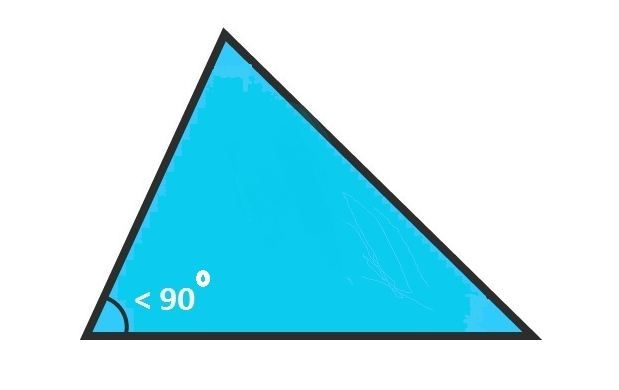Right Angle Triangle

This triangle has any of its one angles to be equal to 90°.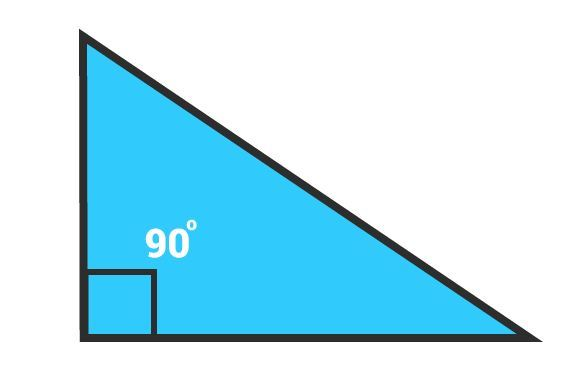Obtuse Angle Triangle

This triangle has any of its one angles more than 90°.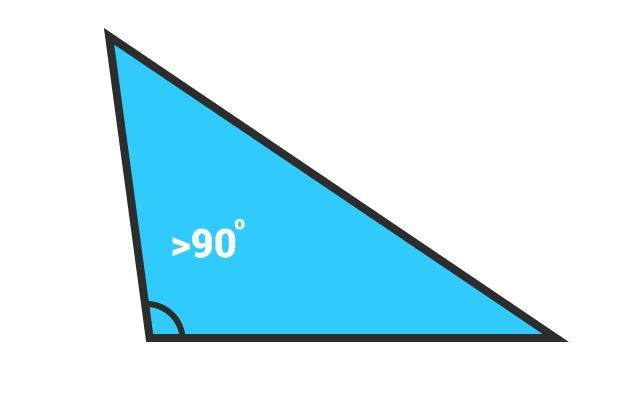Area of a Triangle

The area for a given triangle can be derived with the help of knowing the length of the base and height. Suppose a triangle with base ‘B’ and height ‘H’ is given to us.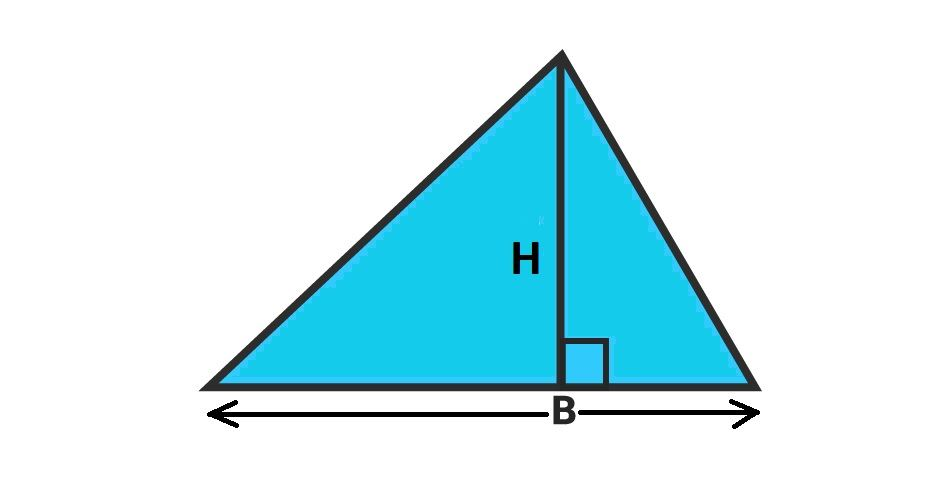The area of a triangle is given by-

Area = 1/2 × Base × Height

Example

Question- Find the area of a triangle having base equal to 9 cm and height equal to 6 cm.

Solution-  We know that Area = 1/2 × Base × Height

= 1/2 × 9 × 6 cm2

= 27 cm2

Heron’s Formula To Find Area of Triangle

Sometimes it is difficult to calculate the height of a triangle, so we cannot use the direct formula.

Therefore Heron’s formula is used to calculate the area by the means of sides of the triangle.

First, e need to calculate the semi perimeter (s).

s = 1/2 (a+b+c),                     (where a,b,c are the three sides of a triangle)

Now Area is given by; A = √s(s-a)(s-b)(s-c)

Example

Question- Find the area of a triangle having sides 5,6 and 7 units length.

Solution-  Using Heron’s formula to find the area of a triangle-

Semiperimeter (s) = 1/2 (a+b+c)

s =  1/2(5 + 6 +7)

s = 9

Now Area \(\bigtriangleup = √s(s-a)(s-b)(s-c)

=√9(9-5)(9-6)(9-7)

=√ 9 × 4 × 3 × 2

=√ 3 × 3 × 2 × 2 × 3 × 2

= 6√6 square units.

Video LessonStay connected with BYJU’S -The Learning App to know more on Maths related topics.